# Chapter4001 and 4002 traingles

11 Dec 2014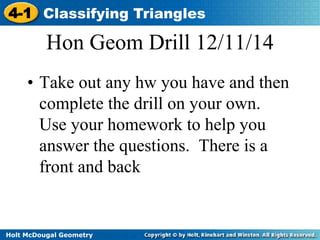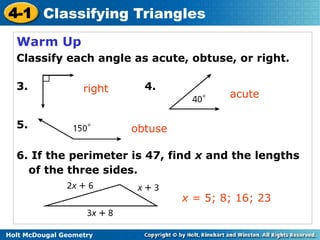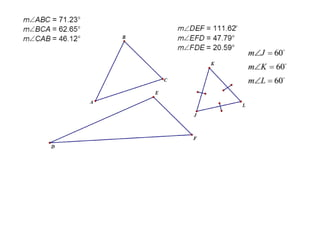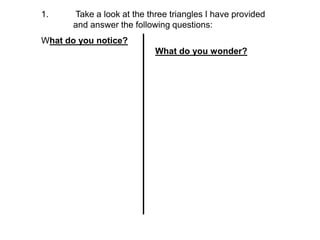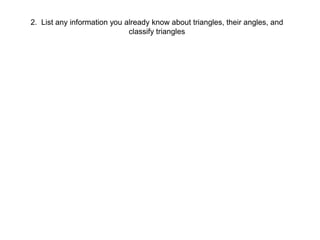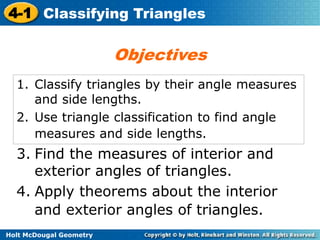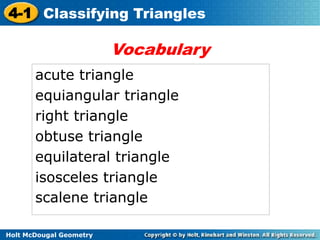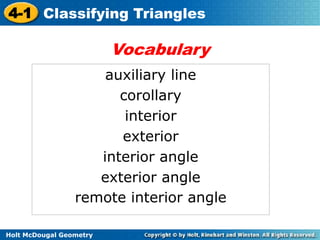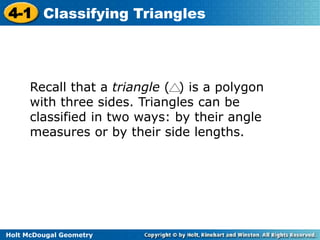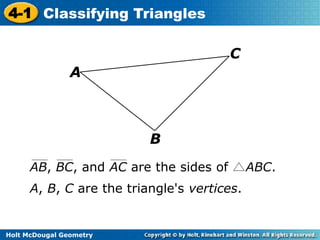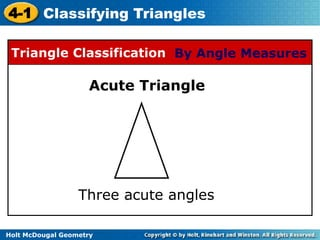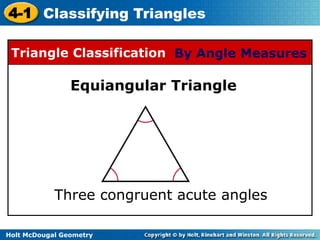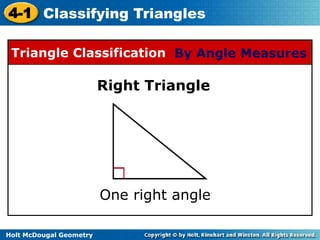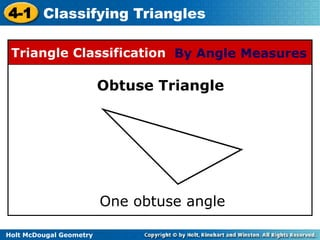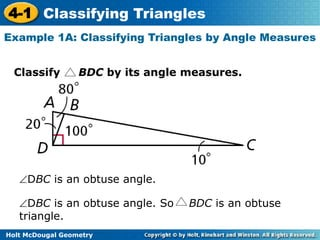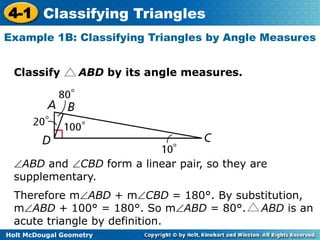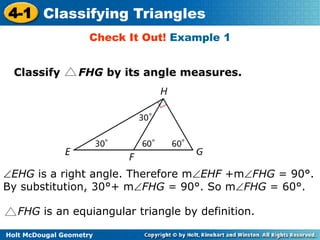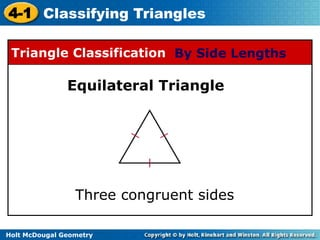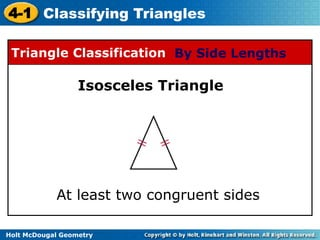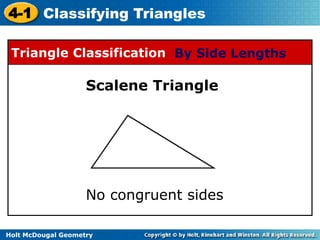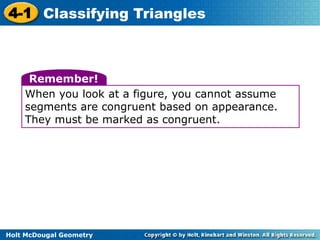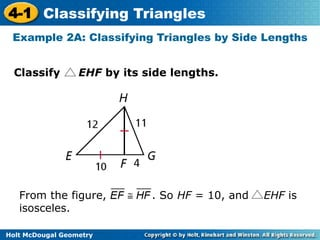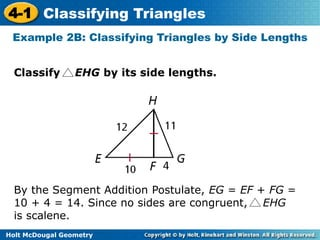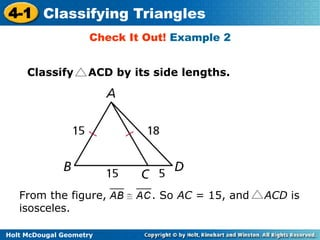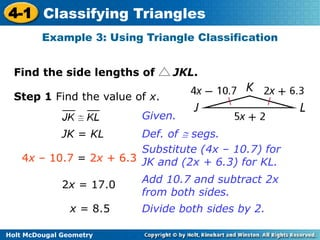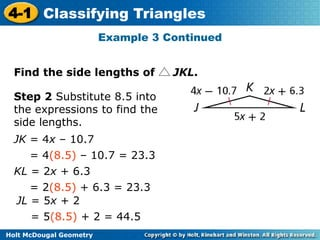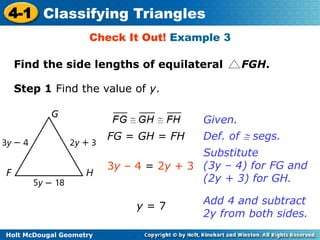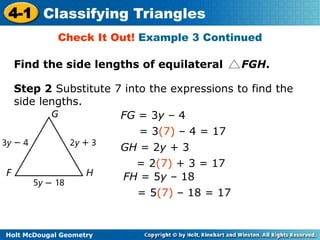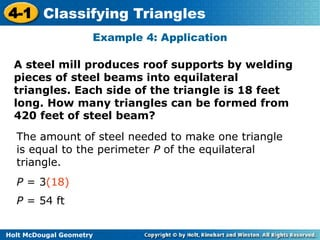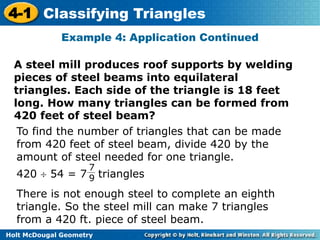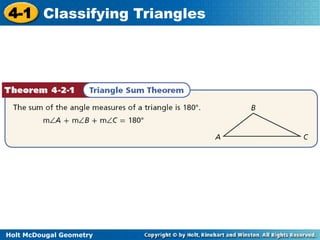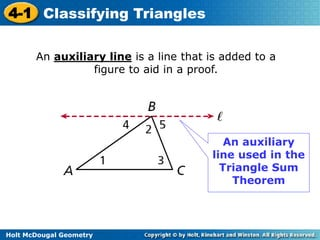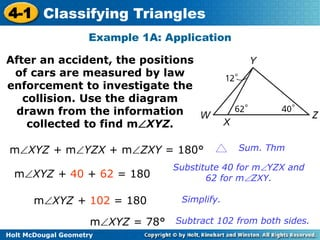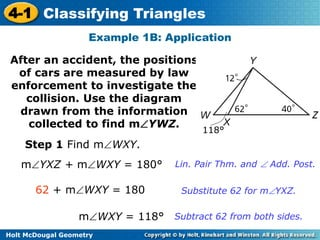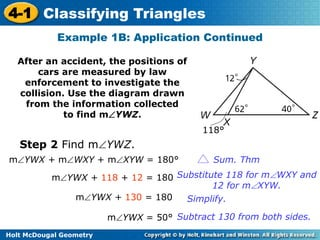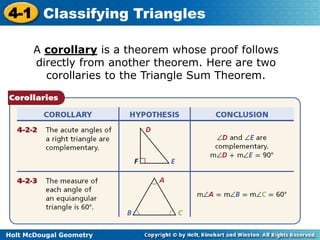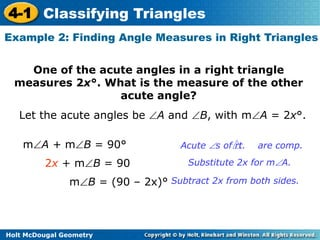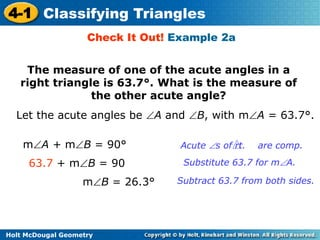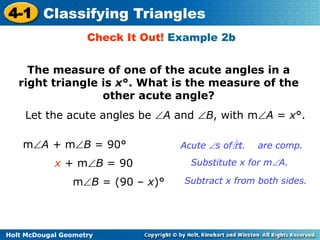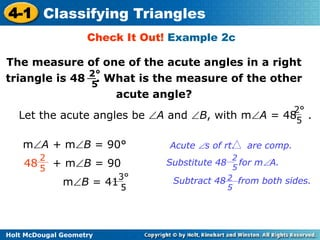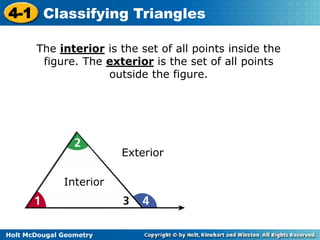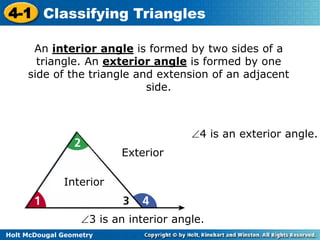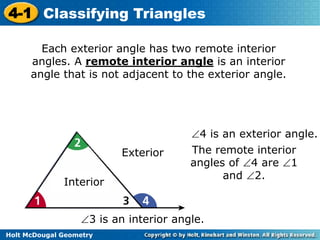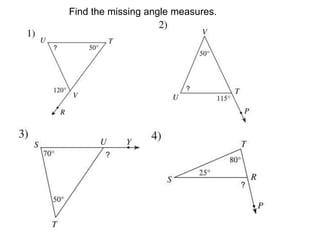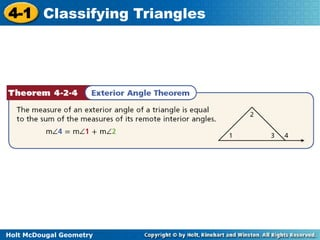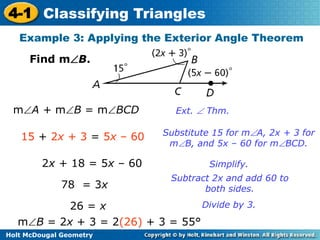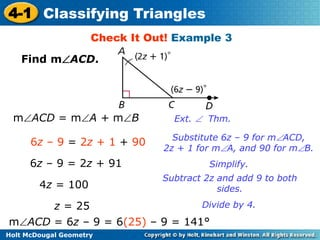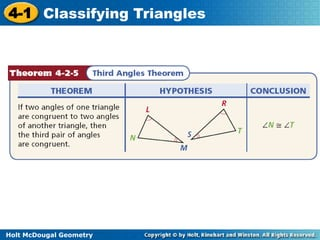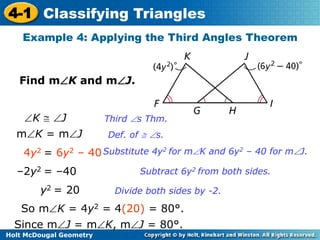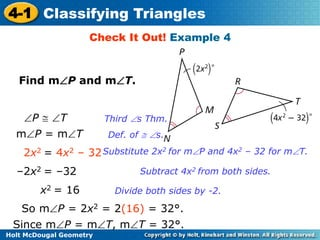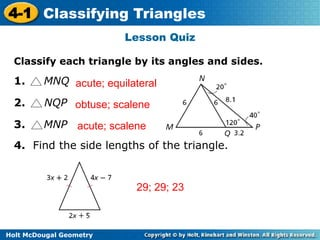1 sur 51

### Chapter4001 and 4002 traingles

• 1. 4-1 Classifying Triangles Hon Geom Drill 12/11/14 • Take out any hw you have and then complete the drill on your own. Use your homework to help you answer the questions. There is a front and back Holt McDougal Geometry
• 2. 4-1 Classifying Triangles Warm Up Classify each angle as acute, obtuse, or right. 3. 4. 5. right 6. If the perimeter is 47, find x and the lengths of the three sides. Holt McDougal Geometry acute x = 5; 8; 16; 23 obtuse
• 4. 1. Take a look at the three triangles I have provided and answer the following questions: What do you notice? What do you wonder?
• 5. 2. List any information you already know about triangles, their angles, and classify triangles
• 6. 4-1 Classifying Triangles 1. Classify triangles by their angle measures and side lengths. 2. Use triangle classification to find angle measures and side lengths. 3. Find the measures of interior and exterior angles of triangles. 4. Apply theorems about the interior and exterior angles of triangles. Holt McDougal Geometry Objectives
• 7. 4-1 Classifying Triangles acute triangle equiangular triangle right triangle obtuse triangle equilateral triangle isosceles triangle scalene triangle Holt McDougal Geometry Vocabulary
• 8. 4-1 Classifying Triangles Holt McDougal Geometry Vocabulary auxiliary line corollary interior exterior interior angle exterior angle remote interior angle
• 9. 4-1 Classifying Triangles Recall that a triangle ( ) is a polygon with three sides. Triangles can be classified in two ways: by their angle measures or by their side lengths. Holt McDougal Geometry
• 10. 4-1 Classifying Triangles Holt McDougal Geometry B A C AB, BC, and AC are the sides of ABC. A, B, C are the triangle's vertices.
• 11. 4-1 Classifying Triangles Triangle Classification By Angle Measures Acute Triangle Three acute angles Holt McDougal Geometry
• 12. 4-1 Classifying Triangles Triangle Classification By Angle Measures Equiangular Triangle Three congruent acute angles Holt McDougal Geometry
• 13. 4-1 Classifying Triangles Triangle Classification By Angle Measures Holt McDougal Geometry Right Triangle One right angle
• 14. 4-1 Classifying Triangles Triangle Classification By Angle Measures Holt McDougal Geometry Obtuse Triangle One obtuse angle
• 15. 4-1 Classifying Triangles Example 1A: Classifying Triangles by Angle Measures Classify BDC by its angle measures. DBC is an obtuse angle. DBC is an obtuse angle. So BDC is an obtuse triangle. Holt McDougal Geometry
• 16. 4-1 Classifying Triangles Example 1B: Classifying Triangles by Angle Measures Classify ABD by its angle measures. ABD and CBD form a linear pair, so they are supplementary. Therefore mABD + mCBD = 180°. By substitution, mABD + 100° = 180°. So mABD = 80°. ABD is an acute triangle by definition. Holt McDougal Geometry
• 17. 4-1 Classifying Triangles Check It Out! Example 1 Classify FHG by its angle measures. EHG is a right angle. Therefore mEHF +mFHG = 90°. By substitution, 30°+ mFHG = 90°. So mFHG = 60°. FHG is an equiangular triangle by definition. Holt McDougal Geometry
• 18. 4-1 Classifying Triangles Triangle Classification By Side Lengths Equilateral Triangle Three congruent sides Holt McDougal Geometry
• 19. 4-1 Classifying Triangles Triangle Classification By Side Lengths Isosceles Triangle At least two congruent sides Holt McDougal Geometry
• 20. 4-1 Classifying Triangles Triangle Classification By Side Lengths Scalene Triangle No congruent sides Holt McDougal Geometry
• 21. 4-1 Classifying Triangles Remember! When you look at a figure, you cannot assume segments are congruent based on appearance. They must be marked as congruent. Holt McDougal Geometry
• 22. 4-1 Classifying Triangles Example 2A: Classifying Triangles by Side Lengths Classify EHF by its side lengths. From the figure, . So HF = 10, and EHF is isosceles. Holt McDougal Geometry
• 23. 4-1 Classifying Triangles Example 2B: Classifying Triangles by Side Lengths Classify EHG by its side lengths. By the Segment Addition Postulate, EG = EF + FG = 10 + 4 = 14. Since no sides are congruent, EHG is scalene. Holt McDougal Geometry
• 24. 4-1 Classifying Triangles Check It Out! Example 2 Classify ACD by its side lengths. From the figure, . So AC = 15, and ACD is isosceles. Holt McDougal Geometry
• 25. 4-1 Classifying Triangles Example 3: Using Triangle Classification Find the side lengths of JKL. Step 1 Find the value of x. Holt McDougal Geometry Given. JK = KL Def. of  segs. 4x – 10.7 = 2x + 6.3 Substitute (4x – 10.7) for JK and (2x + 6.3) for KL. 2x = 17.0 x = 8.5 Add 10.7 and subtract 2x from both sides. Divide both sides by 2.
• 26. 4-1 Classifying Triangles Find the side lengths of JKL. Holt McDougal Geometry Example 3 Continued Step 2 Substitute 8.5 into the expressions to find the side lengths. JK = 4x – 10.7 = 4(8.5) – 10.7 = 23.3 KL = 2x + 6.3 = 2(8.5) + 6.3 = 23.3 JL = 5x + 2 = 5(8.5) + 2 = 44.5
• 27. 4-1 Classifying Triangles Check It Out! Example 3 Find the side lengths of equilateral FGH. Step 1 Find the value of y. Holt McDougal Geometry Given. FG = GH = FH Def. of  segs. 3y – 4 = 2y + 3 Substitute (3y – 4) for FG and (2y + 3) for GH. y = 7 Add 4 and subtract 2y from both sides.
• 28. 4-1 Classifying Triangles Check It Out! Example 3 Continued Find the side lengths of equilateral FGH. Step 2 Substitute 7 into the expressions to find the side lengths. Holt McDougal Geometry FG = 3y – 4 = 3(7) – 4 = 17 GH = 2y + 3 = 2(7) + 3 = 17 FH = 5y – 18 = 5(7) – 18 = 17
• 29. 4-1 Classifying Triangles Example 4: Application A steel mill produces roof supports by welding pieces of steel beams into equilateral triangles. Each side of the triangle is 18 feet long. How many triangles can be formed from 420 feet of steel beam? The amount of steel needed to make one triangle is equal to the perimeter P of the equilateral triangle. P = 3(18) P = 54 ft Holt McDougal Geometry
• 30. 4-1 Classifying Triangles Example 4: Application Continued A steel mill produces roof supports by welding pieces of steel beams into equilateral triangles. Each side of the triangle is 18 feet long. How many triangles can be formed from 420 feet of steel beam? To find the number of triangles that can be made from 420 feet of steel beam, divide 420 by the amount of steel needed for one triangle. 420  54 = 7 triangles 7 9 There is not enough steel to complete an eighth triangle. So the steel mill can make 7 triangles from a 420 ft. piece of steel beam. Holt McDougal Geometry
• 31. 4-1 Classifying Triangles Holt McDougal Geometry
• 32. 4-1 Classifying Triangles An auxiliary line is a line that is added to a Holt McDougal Geometry figure to aid in a proof. An auxiliary line used in the Triangle Sum Theorem
• 33. 4-1 Classifying Triangles Example 1A: Application After an accident, the positions of cars are measured by law enforcement to investigate the collision. Use the diagram drawn from the information collected to find mXYZ. mXYZ + mYZX + mZXY = 180° Sum. Thm mXYZ + 40 + 62 = 180 Holt McDougal Geometry Substitute 40 for mYZX and 62 for mZXY. mXYZ + 102 = 180 Simplify. mXYZ = 78° Subtract 102 from both sides.
• 34. 4-1 Classifying Triangles Example 1B: Application After an accident, the positions of cars are measured by law enforcement to investigate the collision. Use the diagram drawn from the information collected to find mYWZ. Step 1 Find mWXY. mYXZ + mWXY = 180° Lin. Pair Thm. and  Add. Post. 62 + mWXY = 180 Substitute 62 for mYXZ. mWXY = 118° Subtract 62 from both sides. Holt McDougal Geometry 118°
• 35. 4-1 Classifying Triangles Example 1B: Application Continued After an accident, the positions of cars are measured by law enforcement to investigate the collision. Use the diagram drawn from the information collected to find mYWZ. Step 2 Find mYWZ. Holt McDougal Geometry 118° mYWX + mWXY + mXYW = 180° Sum. Thm mYWX + 118 + 12 = 180 Substitute 118 for mWXY and 12 for mXYW. mYWX + 130 = 180 Simplify. mYWX = 50° Subtract 130 from both sides.
• 36. 4-1 Classifying Triangles A corollary is a theorem whose proof follows directly from another theorem. Here are two corollaries to the Triangle Sum Theorem. Holt McDougal Geometry
• 37. 4-1 Classifying Triangles Example 2: Finding Angle Measures in Right Triangles One of the acute angles in a right triangle measures 2x°. What is the measure of the other Let the acute angles be A and B, with mA = 2x°. Holt McDougal Geometry acute angle? mA + mB = 90° Acute s of rt. are comp. 2x + mB = 90 Substitute 2x for mA. mB = (90 – 2x)° Subtract 2x from both sides.
• 38. 4-1 Classifying Triangles Check It Out! Example 2a The measure of one of the acute angles in a right triangle is 63.7°. What is the measure of the other acute angle? Let the acute angles be A and B, with mA = 63.7°. mA + mB = 90° 63.7 + mB = 90 Substitute 63.7 for mA. mB = 26.3° Subtract 63.7 from both sides. Holt McDougal Geometry Acute s of rt. are comp.
• 39. 4-1 Classifying Triangles Check It Out! Example 2b The measure of one of the acute angles in a right triangle is x°. What is the measure of the Let the acute angles be A and B, with mA = x°. Holt McDougal Geometry other acute angle? mA + mB = 90° Acute s of rt. are comp. x + mB = 90 Substitute x for mA. mB = (90 – x)° Subtract x from both sides.
• 40. 4-1 Classifying Triangles Check It Out! Example 2c The measure of one of the acute angles in a right triangle is 48 . What is the measure of the other 2° 5 Let the acute angles be A and B, with mA = 48 . 2 5 48 + mB = 90 Holt McDougal Geometry acute angle? mA + mB = 90° Acute s of rt. are comp. 2° 5 Substitute 48 for mA. Subtract 48 2 from both sides. 5 2 5 3° 5 mB = 41
• 41. 4-1 Classifying Triangles The interior is the set of all points inside the figure. The exterior is the set of all points Holt McDougal Geometry outside the figure. Interior Exterior
• 42. 4-1 Classifying Triangles An interior angle is formed by two sides of a triangle. An exterior angle is formed by one side of the triangle and extension of an adjacent Holt McDougal Geometry side. Interior Exterior 4 is an exterior angle. 3 is an interior angle.
• 43. 4-1 Classifying Triangles Each exterior angle has two remote interior angles. A remote interior angle is an interior angle that is not adjacent to the exterior angle. Interior Holt McDougal Geometry Exterior 4 is an exterior angle. The remote interior angles of 4 are 1 3 is an interior angle. and 2.
• 44. Find the missing angle measures.
• 45. 4-1 Classifying Triangles Holt McDougal Geometry
• 46. 4-1 Classifying Triangles Example 3: Applying the Exterior Angle Theorem Find mB. mA + mB = mBCD Ext.  Thm. 15 + 2x + 3 = 5x – 60 Substitute 15 for mA, 2x + 3 for Holt McDougal Geometry mB, and 5x – 60 for mBCD. 2x + 18 = 5x – 60 Simplify. 78 = 3x Subtract 2x and add 60 to both sides. 26 = x Divide by 3. mB = 2x + 3 = 2(26) + 3 = 55°
• 47. 4-1 Classifying Triangles Check It Out! Example 3 Find mACD. mACD = mA + mB Ext.  Thm. 6z – 9 = 2z + 1 + 90 Substitute 6z – 9 for mACD, Holt McDougal Geometry 2z + 1 for mA, and 90 for mB. 6z – 9 = 2z + 91 Simplify. 4z = 100 Subtract 2z and add 9 to both sides. z = 25 Divide by 4. mACD = 6z – 9 = 6(25) – 9 = 141°
• 48. 4-1 Classifying Triangles Holt McDougal Geometry
• 49. 4-1 Classifying Triangles Example 4: Applying the Third Angles Theorem Find mK and mJ. K  J mK = mJ 4y2 = 6y2 – 40 –2y2 = –40 y2 = 20 So mK = 4y2 = 4(20) = 80°. Since mJ = mK, mJ = 80°. Holt McDougal Geometry Third s Thm. Def. of  s. Substitute 4y2 for mK and 6y2 – 40 for mJ. Subtract 6y2 from both sides. Divide both sides by -2.
• 50. 4-1 Classifying Triangles Check It Out! Example 4 Find mP and mT. P  T mP = mT 2x2 = 4x2 – 32 –2x2 = –32 x2 = 16 So mP = 2x2 = 2(16) = 32°. Since mP = mT, mT = 32°. Holt McDougal Geometry Third s Thm. Def. of  s. Substitute 2x2 for mP and 4x2 – 32 for mT. Subtract 4x2 from both sides. Divide both sides by -2.
• 51. 4-1 Classifying Triangles acute; equilateral obtuse; scalene acute; scalene Holt McDougal Geometry Lesson Quiz Classify each triangle by its angles and sides. 1. MNQ 2. NQP 3. MNP 4. Find the side lengths of the triangle. 29; 29; 23